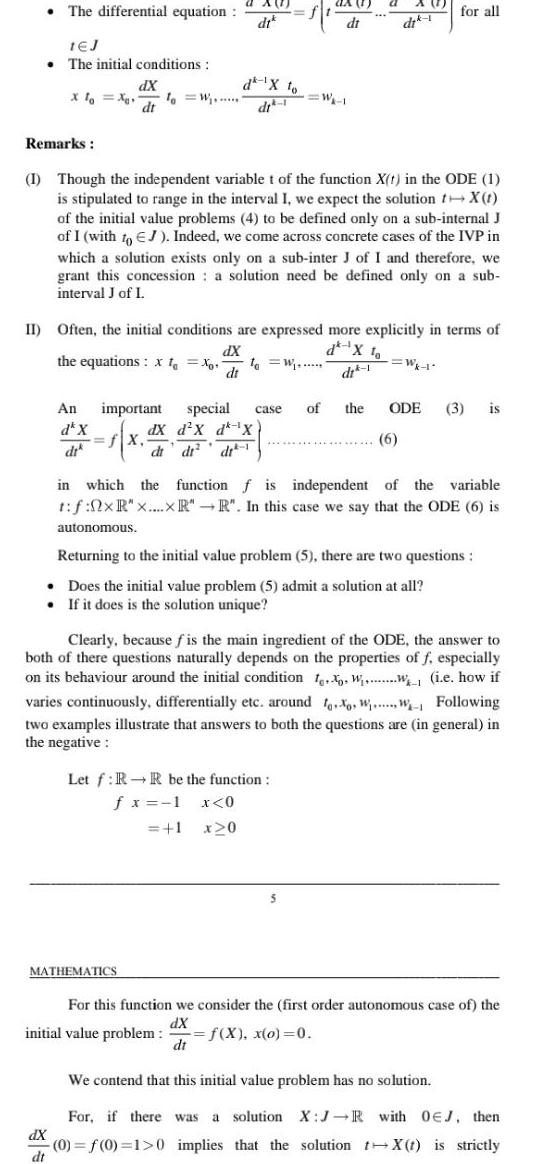Question:

# The differential equation 1EJ The initial conditions Remarks

Last updated: 9/18/2023The differential equation 1EJ The initial conditions Remarks Xlo X dX dt dX dt An da X dr 1 W a A dt f d X to dt 1 MATHEMATICS I Though the independent variable t of the function X t in the ODE 1 is stipulated to range in the interval I we expect the solution t X t of the initial value problems 4 to be defined only on a sub internal J of I with to EJ Indeed we come across concrete cases of the IVP in which a solution exists only on a sub inter J of I and therefore we grant this concession a solution need be defined only on a sub interval J of I II Often the initial conditions are expressed more explicitly in terms of dX the equations xt Xo to W dt da W 1 Let f RR be the function fx 1 x 0 1 x20 di dik 1 for all important special case of the ODE 3 is dX d X d X fx 6 di di di initial value problem f X x o 0 dt in which the function f is independent of the variable t f 0xR x xR R In this case we say that the ODE 6 is autonomous Returning to the initial value problem 5 there are two questions Does the initial value problem 5 admit a solution at all If it does is the solution unique Clearly because fis the main ingredient of the ODE the answer to both of there questions naturally depends on the properties of f especially on its behaviour around the initial condition t WW i e how if varies continuously differentially etc around too WW Following two examples illustrate that answers to both the questions are in general in the negative For this function we consider the first order autonomous case of the dX We contend that this initial value problem has no solution For if there was a solution X J R with OEJ then 0 f 0 1 0 implies that the solution tX t is strictly